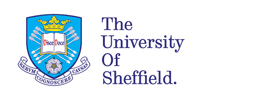# A Bayesian Statistics Reading List

I am frequently asked to recommend books on Bayesian Statistics. Here are a few suggestions, with notes. Note that this page was written in 2004. There are surely many newer and excellent books, but I still think the ones listed here are worth studying.

### Preparatory books

In this category I include some recommendations that are preparatory reading. A very fine book by Dennis Lindley deals with decision making, in very much a Bayesian way using personal probability. And my 1988 book is an introduction to personal probability, which I believe it is important to understand if you are interested in learning about Bayesian Statistics.

Neither book assumes knowledge of mathematics above a very elementary level.

• Lindley, D.V. (1980). Making Decisions, 2nd ed. Wiley, New York.
• O'Hagan, A. (1988). Probability: Methods and Measurement. Chapman and Hall, London.

### Introductory books

The best introductory text at a truly introductory level, with only the most elementary mathematics required, is by Don Berry. It has a slant to medical applications.
• Berry, D.A. (1996). Statistics: A Bayesian Perspective. Duxbury, London.
The best text that is also introductory but assumes college level mathematics is by Peter Lee.
• Lee, P.M. (2004). Bayesian Statistics: An Introduction, 3rd edition, Arnold, London.